Recursive Synthesis of Triangle, Sawtooth and Square waves

First, let's look at the common aspects of digital synthesis. It will help us deduce some formulae. Surely, you know all about the concepts of digital sound, but in spite of this I recommend you not to skip it. So, we have a ceaseless analog signal - perfect and smooth (for example a sine wave):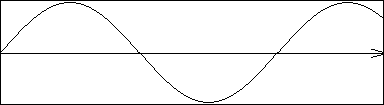Fig. 1

This signal comes from "real life". We should transfer it to the computer world - the world of numbers. How is this done? There is a parameter called discretization frequency. It means that we divide each second into a big amount of parts or samples. The discretization frequency is the number of such parts per second. The value of each sample is simply the voltage of the original signal taken from the corresponding time period and converted by the soundcard's ADC (Analog-to-Digital-Converter) to signed computer words - this is 16-bit recording. That is, we replace the original ceaseless signal with a discrete signal and therefore the higher discretization frequency the better. So, we get a discrete signal: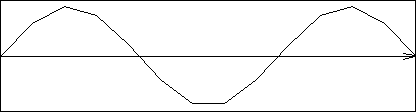Fig. 2

Terrible, isn't it? You can say: "We have never seen such distortions in sound editing programs like Sound Forge or Cool Edit!" Right, because these programs use interpolation to draw the wave on the screen. But this interpolation is only graphical, the actual signal is always discrete as in fig. 2.

Well, we're going on. Now we can come close to the topic of this article. There's a question: can we produce for example a sine wave with a frequency of 6,000 Hz if the discretization frequency will be 11,025 Hz? Answer: no, we can't. A well known Nyquist theorem claims: "If we have a discrete signal with discretization frequency frq then the highest frequency we can store in this signal is maxfrq=frq/2". If we want to synthesize sound with 44,100 samples per second then the highest wave frequency we can produce is 44,100/2=22,050 Hz and so on. But you shouldn't think that a high discretization frequency is required only to produce high frequency waves. Look at Fig. 1 and Fig. 2. If we increase the discretization frequency we will a get signal that differs very little from the original signal. And if this frequency approaches infinity, we'll theoretically get a signal which comes very close to the original.

Now look at the following: (sorry for small pictures)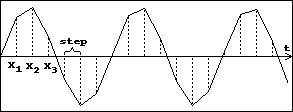Fig. 3

There are some symbols: Xn - these are the values of time when the corresponding sample values are taken. Let's define a function f(x) - it determines the waveform of our sound. In our case f(x)=sin x. Obviously, the samples in the digital signal will be f(X0), f(X1), f(X2), ..., f(Xn)

And now we define a value called step - this is the interval of time between two neighboring samples. If you think a bit, you'll notice that step=1/discretization_frequency.

I think that you see that X1=X0+step, X2=X1+step, ..., Xn=Xn-1+step

This is a recurrence relation, isn't it? But this is not enough to say about recursive synthesis. However, IF WE FIND THAT WE CAN DERIVE f(Xn) FROM SOME AMOUNT OF PREVIOUS SAMPLES (PREFERABLY FROM f(Xn-1)) THEN WE CAN SPEAK ABOUT RECURSIVE SYNTHESIS OF THIS FUNCTION. Derivation means that

f(Xn)=f(Xn-1)+A

`   `
(*) or

f(Xn)=f(Xn-1)*A

`   `
(**) or

a combination of this (***), where A is 1. constant (the best case) or 2. some equation with changing variables. The second case is less preferable than first 'cos we need to calculate this A at every step of calculating f(x).

You might say "This is crap, I should perform multiplication or addition to produce every sample! Using the Look-Up Tables (LUT) is much easier! I should only read a number from an array, not having to calculate it!" But I should tell you: ATTENTION! LOOK-UP TABLES ARE INAPPLICABLE IN SOUND SYNTHESIS!!! You simply receive wrong waves with them! If you are interested why, you can send an e-mail to me and I'll explain this fact to you. Also with LUTs you can't get waves with changing frequencies. Well, you can, but you MUST recalculate LUT for every new frequency! It eats a lot of time. With recursive synthesis changing a wave's frequency is as easy as the fact that you can't live for ever at this Earth.

So, to check the capability of your function to be recursive you should use the following algorithm: (I think that the copyright is mine 'cos I haven't ever seen this)

1. Write down the full formula of your function f(x), for example, f(x)=2x (quite a simple case, isn't it?)

2. Then take the value of your formula not from x but from x+step: f(x+step)=2(x+step)=2x+2step

3. Try to find the way of deriving f(x+step) from f(x) - try to find an equivalent formula which has a form (*) or (**) or (***). In our case we have already found such a formula: 2x+2step, because 2x is a f(x) and 2step is a constant - this is the (*) case. In other formulae, i.e. for cosine or sine, this step is a bit harder, even a big bit harder, so you should know some school and university formulae perfectly. :)

When you have got a recursive formula you can jump and yell, "I've done it!" :) If you have read this article from the beginning you should already have some ideas about how to code this.

If you can't derive such a formula you have two ways:

1. Say "This formula doesn't have a recursive form!"
2. Try to find this form once more. ;-(

[Ed.: Iliks now knows a more intelligent way of doing this. It will be explained in Hugi 23.]

That is the whole algorithm. The following text contains just examples of application of this method in order to synthesize common waveforms. Be sure to read it.

Synthesis of some common waveforms

Uff, yeah! The theoretical part has been done! Now let's look at the short index of the following:

1. Synthesis of sine wave
2. Synthesis of sawtooth wave
3. Synthesis of triangle wave
4. Bonus. Synthesis of square wave
5. Pulse Width Parameter and how to implement it.

Sine wave

Well, I must give a credit. I saw the final formula of the following deduction in Franky's article in Hugi 16 but he didn't prove it at all. He simply said that he deduced it using derivations. Well, I agree with him that (cos wx)'=-w*sin wx, but he simply wrote this statement without proving that sin=sin+wcos. Unlike him, I proved this formula, and there's no need of using derivations at all, more, I have no idea how to prove it with derivations. Franky, if you are reading this article now then please contact me and give me your proof of this, if you have it, of course! So, let's start. We will use my algorithm mentioned above.

We have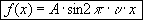where A is the amplitude, or volume of our wave (0-32767), and the symbol after Pi is the frequency of our wave, not the discretization frequency.

If you don't know physics, you can wonder from where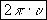appears. Well, there is a concept of cyclic frequency in physics, which shows us how many periods of wave are restricted in the interval of time equal to 2*pi seconds - 6, 28 seconds approximately. I won't explain all this stuff, it will be better to say that if you want to produce a sine wave with the frequency frq then your function should have the following form: f(x)=sin(2Pi*frq*x)

Then we should derive f(x+step) from f(x). Ok, let's do it. I think it's obvious that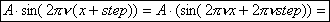Now we should use the following school formula: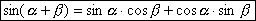We'll get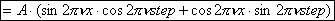Next, we should use more complicated mathematical stuff, the theory of limits. If you don't know what's the hell this means I can't help you - it's quite a big part of math analysis. Simply enter university and you'll know all these things.

For further reasoning we need two limits: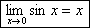(1)

and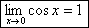(2).

I can deduce this limits but I won't do it because it will take too much space and this is not our main topic. Have you got any idea about how to use these equations in the previous formula? !Tada-dam! This is simple. Somewhere in the beginning of this article I wrote that step=1/discretization_frequency. The discretization frequency is quite a big number and therefore its reciprocal value will be a very small number, very close to zero. And therefore if we multiply any number with this number we will get also a number which is very close to zero. Thus, we can say that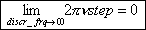And only after this fact we can use limits (1) and (2). So, our final formula will be the following: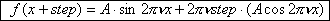or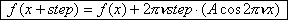We have deduced the recursive formula. However, we see that we Also have to calculate. Which is why we should also deduce one more formula.

But it is very easy after aforecited reasoning. You know that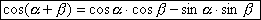We should do the same deductions as for sine. And this is already your job if you wanna see the following proved. I just give the final recursive formula for cosine: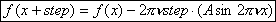We have got all the necessary things to implement this technique In the computer. Next I'll give you a procedure in C that synthesizes a sine wave, but now I want to give you some advice. This algorithm has its own limitations, like all things do. For good fidelity of waveform we should use the calculating features of the computer very carefully, because in the recursive formula any following value of the function depends on the previous ones, so if we do inaccurate calculations we will get poor waveform quality in the end. Thus, just avoid possible loss of data when converting, dividing, multiplying numbers! Meet: The Source.

```void sinus(float frq)
{
float temp=2.0*M_PI*frq/discr_frq;
float cos=16384.0;
float sin=0.0;
short output[length_of_sound];

output=sin;
for (int i=1; i<=length_of_sound-1; ++i)
{
sin+=temp*cos;
cos-=temp*sin;
output[i]=int(0.5+sin);
}
}```

I'm sure you know that int(0.5+sin) means Round(sin). Doubtless, rounding gives us a higher quality waveform. The amplitude of the signal is the first value of cos. The main fact is that you can change the amplitude and the frequency in the inner loop! However, Frankie wrote about it in Hugi 16. All you have to do for this is to recalculate temp according to the changes.

Sawtooth wave

If you don't know what a sawtooth wave looks like then look at the following: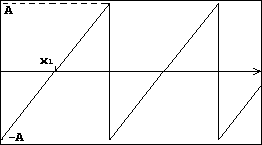NB! The waveform comes at the first onset to minimal level after measuring up to the maximal level, not through the zero! Mathematically, there should be no vertical lines in the graph, but sound editors simply draw lines from the previous point to the following. Let's deduce the recursive formula for the sawtooth function.

I think it is obvious that a sawtooth wave is simply a line. Yep, ingenious things are simple! And all we have to do is to remember the equation of the line. From school you should know that this is the first-order equation and its form is f(x)=kx+b.

Look at the two points: (0;-A) and (x1;0). We see that they belong to our graph. Thus, their coordinates match our equation. Now we ought to solve system of two equations: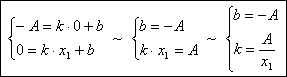We have found that our sawtooth consists of lines that are set by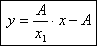equation. Let's find f(x+step).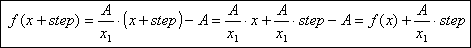Now about the implementation of this. It is obvious that we should have a counter which will count the calculated samples and check whether we have got through the current period of function or not. When we've achieved the minimal value of the saw, we set the value of the current sample to -A (our amplitude) and continue calculating. It's quite clear that x1=T/2 (half-period of our function) and T=1/frq where frq is the frequency of the function. Thus, x1=1/(2*frq). Next, how to calculate whether we get through a period or not? If you think, you'll find that discretization_frequency / frequency_of_wave value is the period of our function but in samples. Catch the point? So, the source is coming.

```void sawtooth(float frq)
{
float temp1=discr_frq/frq;
float temp=2.0*20000/temp1;
float a;
int counter=1;

a=-20000;                                                  //amplitude
output=a;
for (i=1; i<=length_of_sound-1;++i)
{
output[i]=output[i-1]+temp+0.5;
++counter;
if (counter>int(0.5+temp1))                       //   !!!
{
output[i]=a;
counter=0;
}
}
}```

As in the previous example, as well as in the following ones, you can easily change the frequency and the amplitude, all you have to do is to recalculate the dependent variables.

I repeat once more: you MUST be accurate in your calculations! You shouldn't say "Who cares!" There is a bottleneck in the line marked with "!!!". If you don't round the value of temp1 it will be simply truncated. That is why you will get a wrong waveform! However, the distortions are not significant - you won't see them in a sound editor, only in your program which prints the data of the wav file. But this is so only in this concrete case. Who knows what you'll get in other applications if you aren't careful! By the way, experiment with this, check what will be if you don't round temp1!

We're going on!

Triangle wave

I won't draw its waveform - I think these things should be obvious - it is simply a composition of two sawtooth waves. I have explained all this stuff in the previous chapter so you should get outside of this all alone. The maximum I can give you is my source.

```void triangle(float frq)
{
int counter=int(0.5+discr_frq/(4*frq));
output=0;
for (i=1;i<=length_of_sound-1;++i)
{
if (counter<int(0.5+discr_frq/(2*frq)))
{
++counter;
output[i]=output[i1]+int(0.5+(4*20000*frq/float(discr_frq)));
}
else
{
++counter;
if (counter>float(discr_frq)/frq)
{
counter=0;
output[i]=-20000;
} else
output[i]=output[i-1]-int(0.5+(4*20000*frq/float(discr_frq)));
}
}
}```

Square wave

Well, it's just a bonus because it doesn't fit the recursive synthesis principles. The square wave is a composition of two horizontal lines - the first half of the period is a line y=A (A-amplitude) and the second and the last half is a line y=-A. Like with the sawtooth wave there are no null samples at all! Of course if A doesn't equal to zero.

Any further explanation is not necessary. See the program!

```void square(float frq)
{
float temp=discr_frq/frq;
float temp1=temp/2;
int counter=1;

output=20000;

for(int i=1;i<=length_of_sound-1;++i)
{
if (counter<=temp1)
{
output[i]=20000;
++counter;
} else
{
output[i]=-20000;
++counter;
if (counter>temp)
{
counter=0;
}
}
}
}```

Pulse Width Parameter and how to implement it

The Pulse Width parameter (in the following simply PW) is a fractional value between 0 and 1 which defines "proportions" of our wave. As I know, PW is defined for square and triangle waves. The sawtooth wave is just a triangle wave with PW equal to one. For an exact definition of PW look at the pictures.

For square: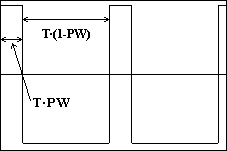For triangle: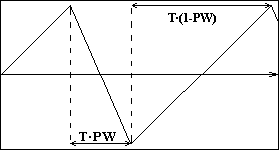where T is a period of our function.

We have to do only minimal changes in our routines so I just give the complete source to you.

1. For square:

```void square(float frq)
{
float pulsewidth=0.2;
float temp=discr_frq/frq;
float temp1=temp*pulsewidth;
int counter=1;

output=20000;

for(int i=1;i<=length_of_sound-1;++i)
{
if (counter<=temp1)
{
output[i]=20000;
++counter;
} else
{
output[i]=-20000;
++counter;
if (counter>temp)
{
counter=0;
}
}
}
}```

2. For triangle:

```void triangle(float frq)
{
float pulsewidth=0.7;
int counter=int(0.5+(pulsewidth*discr_frq)/(2.0*frq));
int temp=int(0.5+(2*20000*frq/(pulsewidth*discr_frq)));
int temp1=int(0.5+(2*20000*frq/((1-pulsewidth)*discr_frq)));
int temp2=int(0.5+float(discr_frq)/frq);
int temp3=int(0.5+(pulsewidth*discr_frq/frq);

output=0;

for (int i=1;i<=length_of_sound-1;++i)
{
if (counter<temp3)
{
++counter;
output[i]=output[i-1]+temp;
}
else
{
++counter;
if (counter>temp2)
{
counter=0;
output[i]=-20000;
} else
output[i]=output[i-1]-temp1
}
}
}```

If you have no ideas how I've done it then simply reread the explanation for the sawtooth wave, then deduce the formulae for the triangle wave and then prove the formulae for the case with pulsewidth. It's really simple!

Now I have found that sine with pulse width isn't impossible. I think I'll do it, however with more complicated ideas! Sure, not tomorrow but maybe in the next issue of Hugi, who knows? :)

In the end of this article I wanna say the following things:

1. If you have read it from the beginning to the end then I congratulate you! Thanks. I'm sure this information won't be useless for you.

2. If you like this article, I can write several more, especially for Hugi. I can light up the following themes: 1) The complete description of the wav format. 2) Wow! Distortion and morphing (like Smorphi does!) 3) Working with DirectSound (beginner's course).

How can you force me to write one of this? Simply send me an email in which you write which one you need the most. If I receive more than 10 emails I'll write the next article with the topic which will have the biggest number of votes. I think that Adok and the other members of the Royal Family won't be against this. Come on! You are welcome!

iliks (Ilja Palopezhencev)
iliks@chat.ru
(preferable)
or iliks@mail.ru